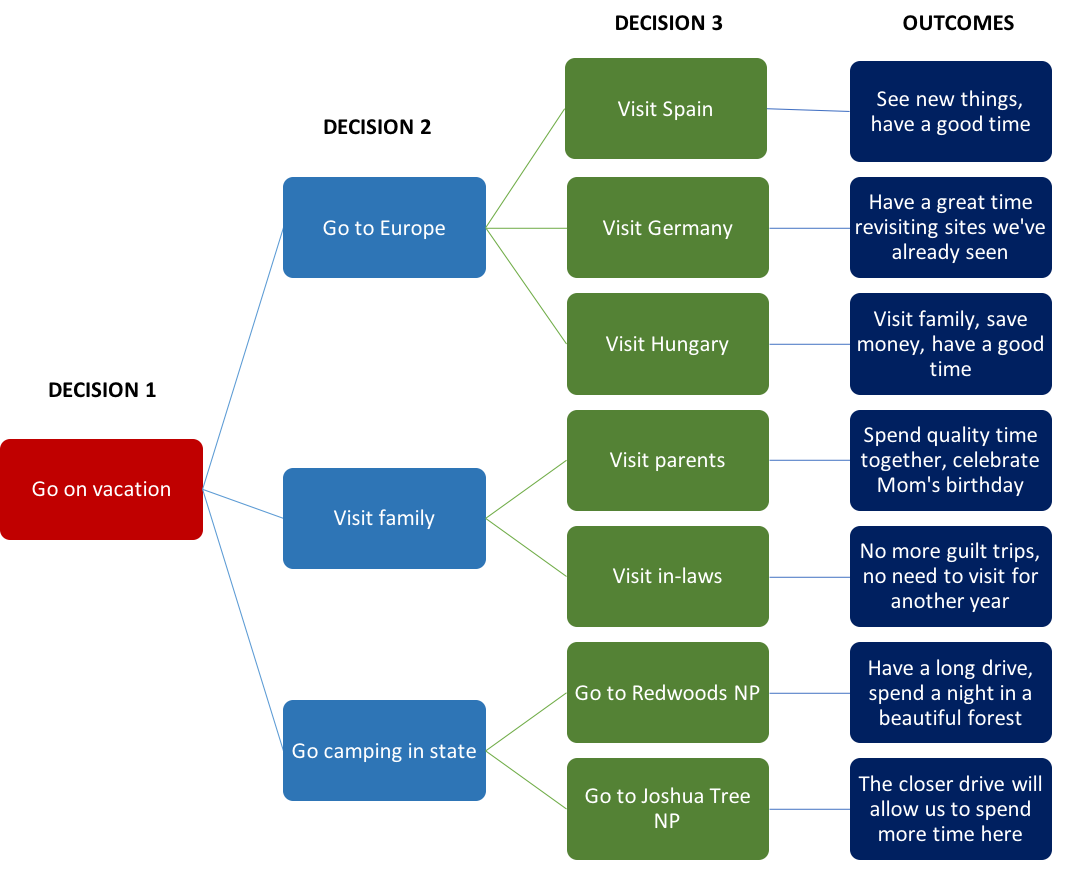## What you’ll learn to do: describe the components and use of a decision tree

Despite the limitations of strict rational decision making, there is no doubt that it still has significant value—especially as we refine and improve our abilities to predict costs and market outcomes for our potential actions. When a business feels it has a reasonably accurate measurement of potential costs and a reasonable prediction of likely future outcomes, that data can be used to calculate the likely value of decision pathways. A useful tool for this is the decision tree, which we are going to learn about now.

### Learning Outcomes

• Describe the components and use of a decision tree.

Decision trees are useful tools, particularly for situations where financial data and probability of outcomes are relatively reliable. They are used to compare the costs and likely values of decision pathways that a business might take. They often include decision alternatives that lead to multiple possible outcomes, with the likelihood of each outcome being measured numerically.

## How to Construct a Decision Tree

A decision tree is a branched flowchart showing multiple pathways for potential decisions and outcomes. The tree starts with what is called a decision node, which signifies that a decision must be made.An example of a decision tree

From the decision node, a branch is created for each of the alternative choices under consideration. The initial decision might lead to another decision, in which case a new decision node is created and new branches are added to show each alternative pathway for the new decision. The result is a series of decision pathways. The flowchart might include only one or two decisions with only one or two alternatives, or it can become a complex sequence of many decisions with many alternatives at each node.

Along the decision pathway, there is usually some point at which a decision leads to an uncertain outcome. That is, a decision could result in multiple possible outcomes, so an uncertainty node is added to the tree at that point. Branches come from that uncertainty node showing the different possible outcomes.

Eventually, each pathway reaches a final outcome. The decision tree, then, is a combination of decision nodes, uncertainty nodes, branches coming from each of these nodes, and final outcomes as the result of the pathways.

## How to Make Calculations with a Decision Tree

Even in only this simple form, a decision tree is useful to show the possibilities for a decision. However, a decision tree becomes especially useful when numerical data is added.

First, each decision usually involves costs. If a company decides to produce a product, engage in market research, advertise, or any other number of activities, the predicted costs for those decisions are written on the appropriate branch of the decision tree. Also, each pathway eventually leads to an outcome that usually results in income. The predicted amount of income provided by each outcome is added to that branch of the decision tree.

The other numerical data that needs to be provided is the probability of each outcome from the uncertainty nodes. If an uncertainty node has two branches that are both equally likely, each should be labeled with a 50 percent, or 0.5, probability. Alternatively, an uncertainty node might have three branches with respective probabilities of 60 percent, 30 percent, and 10 percent. In each case, the total of the percentages at each uncertainty node will be 100 percent, representing all possibilities for that node.

With this numerical data, decision makers can calculate the likely return value for each decision pathway. The value of each final outcome must be multiplied by the probability that the outcome occurs. The total of the possibilities along each branch represent the total predicted value for that decision pathway. The costs involved in that decision pathway must be subtracted to see the final profit that pathway represents.